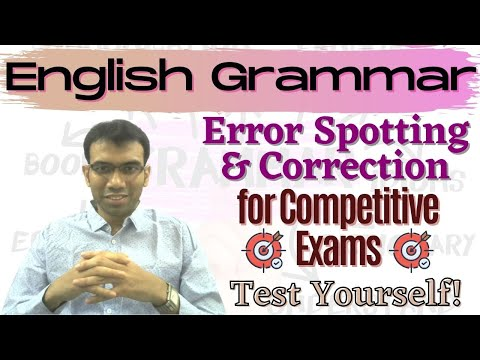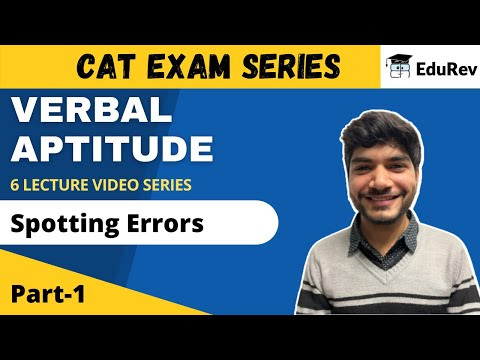Courses

Test: Spotting Errors- 4

30 Questions MCQ Test Verbal Aptitude Basics Duplicated | Test: Spotting Errors- 4

Description
Attempt Test: Spotting Errors- 4 | 30 questions in 40 minutes | Mock test for Banking Exams preparation | Free important questions MCQ to study Verbal Aptitude Basics Duplicated for Banking Exams Exam | Download free PDF with solutions
QUESTION: 1

Solution:

would mind if

QUESTION: 2

Read the each sentence to find out whether there is any grammatical error in it. The error, if any will be in one part of the sentence. The letter of that part is the answer. If there is no error, the answer is 'D'. (Ignore the errors of punctuation, if any).

Solution:

Since the last one month.

QUESTION: 3

Read the each sentence to find out whether there is any grammatical error in it. The error, if any will be in one part of the sentence. The letter of that part is the answer. If there is no error, the answer is 'D'. (Ignore the errors of punctuation, if any).

Solution:

in preparing to face the storm.

QUESTION: 4

Read the each sentence to find out whether there is any grammatical error in it. The error, if any will be in one part of the sentence. The letter of that part is the answer. If there is no error, the answer is 'D'. (Ignore the errors of punctuation, if any).

Solution:

that India has produced

QUESTION: 5

Read each sentence to find out whether there is any grammatical error in it. The error, if any will be in one part of the sentence. The letter of that part is the answer. If there is no error, the answer is 'D'. (Ignore the errors of punctuation, if any).

Solution:

As merry a band of children as I have ever seen since or before.

QUESTION: 6

Read the each sentence to find out whether there is any grammatical error in it. The error, if any will be in one part of the sentence. The letter of that part is the answer. If there is no error, the answer is 'D'. (Ignore the errors of punctuation, if any).

Solution:

to take interest in his school

QUESTION: 7

Read the each sentence to find out whether there is any grammatical error in it. The error, if any will be in one part of the sentence. The letter of that part is the answer. If there is no error, the answer is 'D'. (Ignore the errors of punctuation, if any).

Solution:

Much as I admire him for his sterling qualities.

QUESTION: 8

Read the each sentence to find out whether there is any grammatical error in it. The error, if any will be in one part of the sentence. The letter of that part is the answer. If there is no error, the answer is 'D'. (Ignore the errors of punctuation, if any).

Solution:

Twice twelve make twenty-four

QUESTION: 9

Read the each sentence to find out whether there is any grammatical error in it. The error, if any will be in one part of the sentence. The letter of that part is the answer. If there is no error, the answer is 'D'. (Ignore the errors of punctuation, if any).

Solution:

There is no error in the given sentence.

QUESTION: 10

Read the each sentence to find out whether there is any grammatical error in it. The error, if any will be in one part of the sentence. The letter of that part is the answer. If there is no error, the answer is 'D'. (Ignore the errors of punctuation, if any).

Solution:

The reason why Ram

QUESTION: 11

Read the each sentence to find out whether there is any grammatical error in it. The error, if any will be in one part of the sentence. The letter of that part is the answer. If there is no error, the answer is 'D'. (Ignore the errors of punctuation, if any).

Solution:

The custom still prevails among some groups in India.

QUESTION: 12

Read the each sentence to find out whether there is any grammatical error in it. The error, if any will be in one part of the sentence. The letter of that part is the answer. If there is no error, the answer is 'D'. (Ignore the errors of punctuation, if any).

Solution:

In option ( b) there is an error.

Instead of  ,what  I want is that;

"  I want that "  Should be used.

QUESTION: 13

Read each sentence to find out whether there is any grammatical error in it. The error, if any will be in one part of the sentence. The letter of that part is the answer. If there is no error, the answer is 'D'. (Ignore the errors of punctuation, if any).

Solution:

'how she could kill the wolf'

QUESTION: 14

Read the each sentence to find out whether there is any grammatical error in it. The error, if any will be in one part of the sentence. The letter of that part is the answer. If there is no error, the answer is 'D'. (Ignore the errors of punctuation, if any).

Solution:

QUESTION: 15

Read the each sentence to find out whether there is any grammatical error in it. The error, if any will be in one part of the sentence. The letter of that part is the answer. If there is no error, the answer is 'D'. (Ignore the errors of punctuation, if any).

Solution:

he was looked after by his aunt

QUESTION: 16

Read the each sentence to find out whether there is any grammatical error in it. The error, if any will be in one part of the sentence. The letter of that part is the answer. If there is no error, the answer is 'D'. (Ignore the errors of punctuation, if any).

Solution:

Troy was taken by the Greeks.

QUESTION: 17

Read the each sentence to find out whether there is any grammatical error in it. The error, if any will be in one part of the sentence. The letter of that part is the answer. If there is no error, the answer is 'D'. (Ignore the errors of punctuation, if any).

Solution:

QUESTION: 18

Read the each sentence to find out whether there is any grammatical error in it. The error, if any will be in one part of the sentence. The letter of that part is the answer. If there is no error, the answer is 'D'. (Ignore the errors of punctuation, if any).

Solution:

While he was walking slowly in the park.

QUESTION: 19

Read the each sentence to find out whether there is any grammatical error in it. The error, if any will be in one part of the sentence. The letter of that part is the answer. If there is no error, the answer is 'D'. (Ignore the errors of punctuation, if any).

Solution:

At the present juncture.

QUESTION: 20

Read the each sentence to find out whether there is any grammatical error in it. The error, if any will be in one part of the sentence. The letter of that part is the answer. If there is no error, the answer is 'D'. (Ignore the errors of punctuation, if any).

Solution:

what a science of mental life should be

QUESTION: 21

Read the each sentence to find out whether there is any grammatical error in it. The error, if any will be in one part of the sentence. The letter of that part is the answer. If there is no error, the answer is 'D'. (Ignore the errors of punctuation, if any).

Solution:

If something is permanent it is static.
ex: Lions live in the jungle
If something is temporary it is dynamic in nature
ex: A boy fell into the well.

So, we use into in the given sentence.

QUESTION: 22

Read the each sentence to find out whether there is any grammatical error in it. The error, if any will be in one part of the sentence. The letter of that part is the answer. If there is no error, the answer is 'D'. (Ignore the errors of punctuation, if any).

Solution:

There is no error in the sentence.

QUESTION: 23

Read the each sentence to find out whether there is any grammatical error in it. The error, if any will be in one part of the sentence. The letter of that part is the answer. If there is no error, the answer is 'D'. (Ignore the errors of punctuation, if any).

Solution:

All the furniture have been

QUESTION: 24

Read the each sentence to find out whether there is any grammatical error in it. The error, if any will be in one part of the sentence. The letter of that part is the answer. If there is no error, the answer is 'D'. (Ignore the errors of punctuation, if any).

Solution:

'and he has to see a doctor'

QUESTION: 25

Read the each sentence to find out whether there is any grammatical error in it. The error, if any will be in one part of the sentence. The letter of that part is the answer. If there is no error, the answer is 'D'. (Ignore the errors of punctuation, if any).

Solution:

we should accept all our people as equal

QUESTION: 26

Read the each sentence to find out whether there is any grammatical error in it. The error, if any will be in one part of the sentence. The letter of that part is the answer. If there is no error, the answer is 'D'. (Ignore the errors of punctuation, if any).

Solution:

know whom you want

QUESTION: 27

Read the each sentence to find out whether there is any grammatical error in it. The error, if any will be in one part of the sentence. The letter of that part is the answer. If there is no error, the answer is 'D'. (Ignore the errors of punctuation, if any).

Solution:

his father is junior to

QUESTION: 28

Read the each sentence to find out whether there is any grammatical error in it. The error, if any will be in one part of the sentence. The letter of that part is the answer. If there is no error, the answer is 'D'. (Ignore the errors of punctuation, if any).

Solution:

that the dispute on this issue is between my brother and me

QUESTION: 29

Read the each sentence to find out whether there is any grammatical error in it. The error, if any will be in one part of the sentence. The letter of that part is the answer. If there is no error, the answer is 'D'. (Ignore the errors of punctuation, if any).

Solution:

option ( c) than butter is the correct answer.

Explanation:-  option ( c) is the correct answer because,  with prefer, generally to is used not than.

Similarly with junior ,senior  to is used instead of than.

*Multiple options can be correct
QUESTION: 30

Read the each sentence to find out whether there is any grammatical error in it. The error, if any will be in one part of the sentence. The letter of that part is the answer. If there is no error, the answer is 'D'. (Ignore the errors of punctuation, if any).

Solution:

In option ( b) there is an error, it should be like :-  he has not so far sent. And in the option ( c) ,there  is also error ; it should be like:-  any reply to my letters.Use Code STAYHOME200 and get INR 200 additional OFF Use Coupon Code

Track your progress, build streaks, highlight & save important lessons and more!

Similar ContentRelated tests